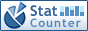Home PC Games Linux Windows Database Network Programming Server Mobile
 Home \ Programming \ Sort search algorithm Java - application examples with recursive dichotomy - TeamCity continuous integration in the Linux installation (Linux) - OS X CAOpenGLLayer how to enable OpenGL 3.2 core profile (Programming) - Install snort intrusion detection system on Debian (Linux) - Smack 4.1.x Upgrade Guide (Linux) - 10 really interesting Linux command (Linux) - Use in Linux ipmitool tool (Linux) - Linux operating system security tools of the Logs (Linux) - Boost notes --Asio - (1) a simple small example of synchronous communication (Programming) - HTML5 Application Cache (Programming) - How to determine whether the Linux server was hacked (Linux) - Linux Open coredump (Linux) - Windows7 system using Vagrant to build Linux virtualized development environment (Linux) - Depth understanding of C language (Programming) - How do I switch from NetworkManager to systemd-network on Linux (Linux) - Spring classic face questions Share (Programming) - CentOS 6.x and CentOS7 installation RPMforge (Linux) - Java study notes: String (Programming) - How to use the TF / SD card making Exynos 4412 u-boot boot disk in Ubuntu (Linux) - Bug tracking library after FastJson omitted the decimal point 0 (Programming) - ElasticSearch basic usage and cluster structures (Server) Sort search algorithm Java - application examples with recursive dichotomy Add Date : 2017-07-22 Problem Description: To find and sort the array elements, and the use of recursive dichotomy. Complete Example 1: public class SortDemo {   public static void main (String [] args)   {       int [] arr = {10,2,300,41,15,6};       for (int a: arr)       {         System.out.print ( "[" + a + "]");       }     // New SortDemo () insertSort (arr).;     new SortDemo () binaryInsertSort (arr).;       System.out.println ();       for (int a: arr)       {         System.out.print ( "[" + a + "]");       }   }        // Sort dichotomy   public void binaryInsertSort (int [] a)   {       for (int i = 1; i < a.length; i ++)       {         int temp = a [i];         int low = 0;         int high = i-1;         while (low < = high)         {             int mid = (low + high) / 2;             if (a [mid] < temp)             {                 low = mid + 1;             }             else             {                 high = mid-1;             }         }         for (int j = i-1; j> = low; j--)         {             a [j + 1] = a [j];         }         a [low] = temp;       }   }        // Insertion sort   public void insertSort (int [] a)   {       Coordinate preceding element // participate compare elements; int i       // Current element involved in the comparison; int key       for (int j = 1; j < a.length; j ++)       {         key = a [j];         i = j-1;         while (i> = 0 && a [i]> key)         {             a [i + 1] = a [i]; // right one             i -; // i advance         }         a [i + 1] = key;       }   } } Complete Example 2: // Binary search import java.util *.; public class BinarySearchDemo {   public static void main (String [] args)   {         int [] arr = {10,2,300,41,15,6};         Arrays.sort (arr); // sort the array of natural         . //System.out.println(new BinarySearchDemo () method1 (arr, 41));         System.out.println (new BinarySearchDemo () method2 (arr.length-1,0, arr, 41).);   }   // Recursive binary search   public String method2 (int b, int s, int [] a, int g)   {       int m; // Find the coordinates of the median part       if (s < b)       {         m = (b + s) / 2;         if (a [m] == g)         {             return "you are looking for [" + g + "] is ranked in the array size [" + (m + 1) + "] position.";         }         else if (a [m]> g) // if the intermediate value is greater than the target value, comparing the left half,         {             b = m-1; // first part of the highest-coordinate values ​​into the middle to find a             return method2 (b, s, a, g);         }                   else // If the intermediate value is smaller than the target value, then the right half of the comparison         {               s = m + 1; // Find the lowest position after part becomes a middle value               return method2 (b, s, a, g);         }       }       return "you are looking for [" + g + "] is not within the array.";   }        // Parameter is an array and the target value   public String method1 (int [] a, int g)   {       Arrays.sort (a); // sort the array of natural       int b = a.length-1; // Find the highest part of Coordinates       int s = 0; // Find the lowest part of Coordinates       int m; // Find the coordinates of the median part       while (b> = s)       {         m = (b + s) / 2;         if (a [m] == g)         {                            return "you are looking for [" + g + "] is ranked in the array size [" + (m + 1) + "] position.";         }         else if (a [m]> g) // if the intermediate value is greater than the target value, comparing the left half,         {             b = m-1; // first part of the highest-coordinate values ​​into the middle to find a         }         else // If the intermediate value is smaller than the target value, then the right half of the comparison         {               s = m + 1; // Find the lowest position after part becomes a middle value         }       }       return "you are looking for [" + g + "] is not within the array.";   }      } More:
 CopyRight 2002-2022 newfreesoft.com, All Rights Reserved.